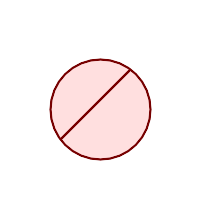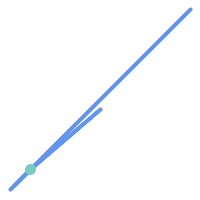## Name

ST_Split — Returns a collection of geometries created by splitting a geometry by another geometry.

## Synopsis

`geometry ST_Split(`geometry input, geometry blade`)`;

## Description

The function supports splitting a LineString by a (Multi)Point, (Multi)LineString or (Multi)Polygon boundary, or a (Multi)Polygon by a LineString. When a (Multi)Polygon is used as as the blade, its linear components (the boundary) are used for splitting the input. The result geometry is always a collection.

This function is in a sense the opposite of ST_Union. Applying ST_Union to the returned collection should theoretically yield the original geometry (although due to numerical rounding this may not be exactly the case).If the the input and blade do not intersect due to numerical precision issues, the input may not be split as expected. To avoid this situation it may be necessary to snap the input to the blade first, using ST_Snap with a small tolerance.

Availability: 2.0.0 requires GEOS

Enhanced: 2.2.0 support for splitting a line by a multiline, a multipoint or (multi)polygon boundary was introduced.

Enhanced: 2.5.0 support for splitting a polygon by a multiline was introduced.

## Examples

Polygon split by a LineBefore SplitAfter split
```SELECT ST_AsText( ST_Split(
ST_Buffer(ST_GeomFromText('POINT(100 90)'), 50), -- circle
ST_MakeLine(ST_Point(10, 10),ST_Point(190, 190)) -- line
));

-- result --
GEOMETRYCOLLECTION(
POLYGON((150 90,149.039264020162 80.2454838991936,146.193976625564 70.8658283817455,..),
POLYGON(..))
)
```

MultiLineString split by a Point, where the point lies exactly on both LineStrings.Before SplitAfter split
```SELECT ST_AsText(ST_Split(
'MULTILINESTRING((10 10, 190 191), (15 15, 30 30, 100 90))',
ST_Point(30,30))) As split;

split
------
GEOMETRYCOLLECTION(
LINESTRING(10 10,30 30),
LINESTRING(30 30,190 190),
LINESTRING(15 15,30 30),
LINESTRING(30 30,100 90)
)
```

LineString split by a Point, where the point does not lie exactly on the line. Shows using ST_Snap to snap the line to the point to allow it to be split.

```WITH data AS (SELECT
'LINESTRING(0 0, 100 100)'::geometry AS line,
'POINT(51 50)':: geometry AS point
)
SELECT ST_AsText( ST_Split(line, point)) AS no_split,
ST_AsText( ST_Split( ST_Snap(line, point, 1), point)) AS split
FROM data;

no_split                   |                                split
---------------------------------------------+---------------------------------------------------------------------
GEOMETRYCOLLECTION(LINESTRING(0 0,100 100)) | GEOMETRYCOLLECTION(LINESTRING(0 0,51 50),LINESTRING(51 50,100 100))

```